Collision Question Question submitted by (16 July 1999)Return to The Archives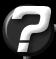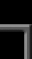I'm writing collision routines for a very simple game, and need to determine the outcome of a collision of two spherical objects, both of which are the same size. One of the objects will remain stationary, and the other is in motion. I have calculated the vector between the two objects, and have a vector of the direction of motion for the moving object. Is there an easy way to calculate the new direction of the moving object?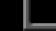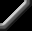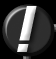let P be the point of contact on the stationary sphere. the vector from the centre of this sphere toward P is PO. the tangent at P is perpendicular to PO. also, suppose the direction of motion of the moving sphere is labeled D.
 ``` \ D \ \ \ \ -----P-------- tangent on the stationary sphere at P | | | | | PO | ```

the moving sphere will bounce away from P along the direction D' such that the angle between D and PO is the same as the angle between D' and PO.

 ``` \ D / D' \ / \ / \ / \ / -----P-------- tangent on the stationary sphere at P | | | | | PO | ```

extending D "into" the sphere and projecting the endpoint onto the tangent yields the vector XP, the component of D' parallel to the tangent.

 ``` \ D / D' \ / \ / \ / \ / -----P----X--- tangent on the stationary sphere at P |\ | | \ | | \ | | \| | PO | ```

of course, if the vectors D and PO are colinear, the moving sphere will simply bounce back in the direction of -kD where k is some constant.Response provided by Hin JangThis article was originally an entry in flipCode's Fountain of Knowledge, an open Question and Answer column that no longer exists.